# 🌈 Graphics¶

## Drawing¶

`screen.``fill`(color)

Fills the screen with the specified `color`.

The color option can be specified using a name (e.g. ‘white’, ‘black’), or an RGB triple.

Example: fill the screen with white
```screen.fill(color='white')
```
Example: fill the screen with red
```screen.fill(color=(255, 0, 0))
```
`screen.``text`(string…, xy=…, color=…, align=…, font=…, font_size=…, max_width=…, max_lines=…, max_height=…)

Draws text `string`.

`xy` is the position that the text will be drawn.

The align option is one of:

topleft, left, bottomleft, top, center, bottom, topright, right, bottomright

If a custom font is used, it must be included in the tingapp bundle.

Example: Write ‘Hello world’ in black on the screen
```screen.text('Hello world!', color='black')
```
Example: changing the alignment
```screen.text('Hello world!', xy=(20,20), color='black', align='topleft')
```
Example: Using a custom font
```screen.text('Hello world!', color='black', font='Helvetica.ttf')
```
Example: Changing the text size
```screen.text('Hello world!', color='black', font_size=50)
```
Example: Confining text to a single line
```screen.text('Lorem ipsum dolor sit amet, consectetur adipiscing elit!', color='black', max_lines=1)
```
Example: Confining text to two lines
```screen.text('Lorem ipsum dolor sit amet, consectetur adipiscing elit!', color='black', max_width=300, max_lines=2)
```
`screen.``rectangle`(xy=…, size=…, color=…, align=…)

Draws a rectangle at position xy, with the specified size and color.

Align is one of

topleft, left, bottomleft, top, center, bottom, topright, right, bottomright,
Example: Drawing a red square
```screen.rectangle(xy=(25,25), size=(100,100), color=(255,0,0))
```
Example: Drawing centered
```screen.rectangle(xy=(160,120), size=(100,100), color=(255,0,0), align='center')
```
`screen.``image`(filename…, xy=…, scale=…, align=…, max_width=…, max_height=…, raise_error=True)

Draws an image with name filename at position xy. If filename is a URL (e.g. http://example.com/cats.png) then it will attempt to download this and display it.

Images can be animated GIFs. Make sure to draw them in a loop() function to see them animate.

Scale is a number that changes the size of the image e.g. scale=2 makes the image bigger, scale=0.5 makes the image smaller. There are also special values ‘fit’ and ‘fill’, which will fit or fill the image according to `max_width` and `max_height`.

Align is one of

topleft, left, bottomleft, top, center, bottom, topright, right, bottomright

If raise_error is True then any errors encountered while opening or retrieving the image will cause an exception. If it is False, then if there is an error a “file not found” icon will be displayed instead

Example: Drawing an Image
```screen.image('tingbot.png', xy=(25,25))
```
Example: Drawing an Image from a URL
```screen.image('http://i.imgur.com/xbT92Gm.png')
```
`screen.``line`(start_xy=…, end_xy=…, color=…, width=…)

Draws a line between `start_xy` and `end_xy`.

## Screen¶

The screen supports all the methods above, and some extras below.

`screen.``update`()

After drawing, this method should to be called to refresh the screen. When drawing in a `draw()` or `loop()` function, this is called automatically, but when drawing in a tight loop, e.g. during a calculation, it can called manually.

Example: An app without a run loop - calling `screen.update()` manually
```import tingbot
from tingbot import *

screen.fill(color='black')

# pump the main run loop just once to make sure the app starts
tingbot.input.EventHandler().poll()

frame_count = 0

while True:
screen.fill(color='black')
screen.text(frame_count)
screen.update()
frame_count += 1
```
`screen.``brightness`

The brightness of the screen, between 0 and 100.

Example: Dimming the screen
```screen.brightness = 25
```
Example: Brightness test app
```import tingbot
from tingbot import *

state = {'brightness': 0}

def loop():
screen.brightness = state['brightness']

screen.fill(color='black')
screen.text('Brightness\n %i' % state['brightness'])

state['brightness'] += 1

if state['brightness'] > 100:
state['brightness'] = 0

tingbot.run(loop)
```

## The `align` option¶

When used without the `xy` parameter, the item is positioned relative to the screen/drawing surface.

Setting Screenshot Code
topleft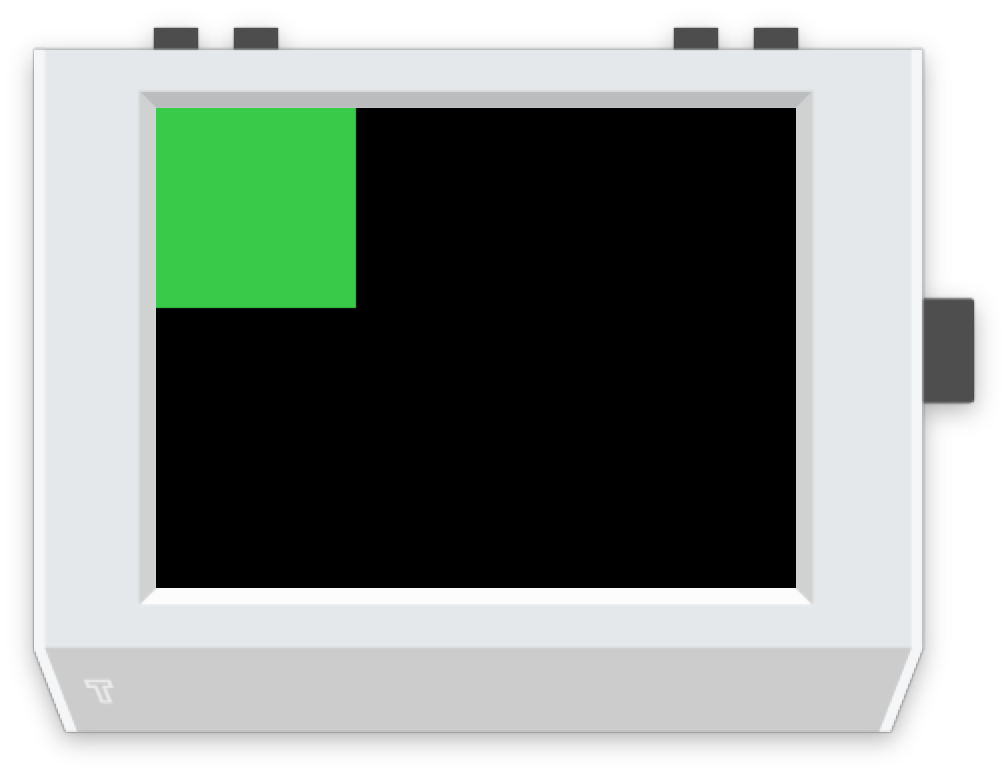`screen.rectangle(color='green', align='topleft')`
top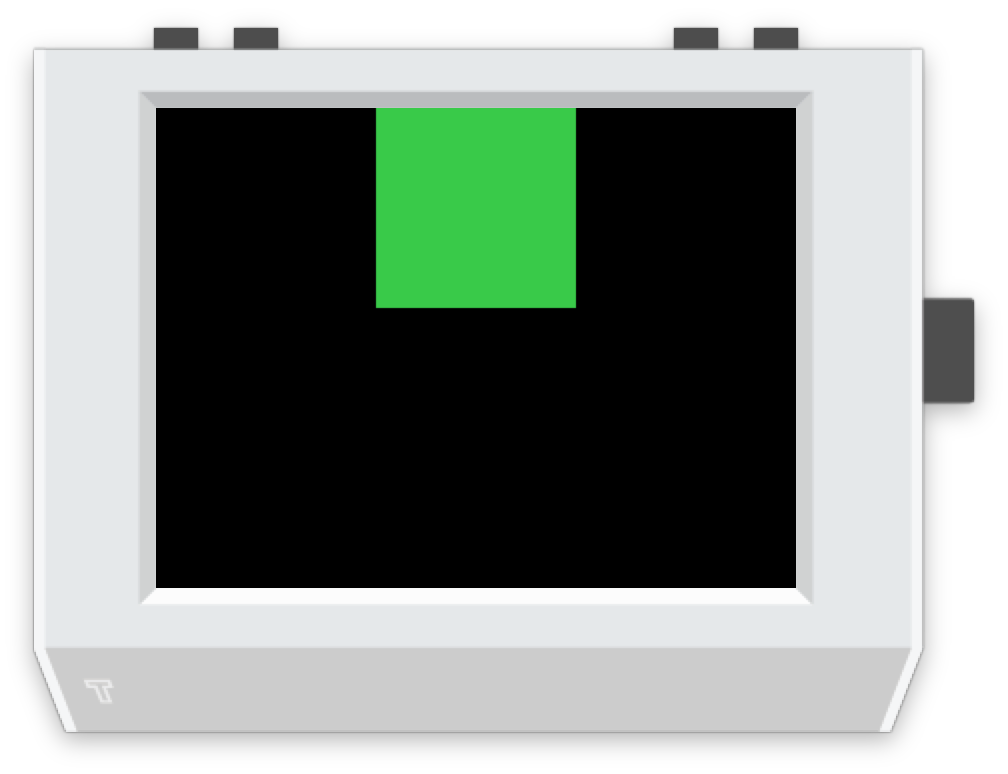`screen.rectangle(color='green', align='top')`
topright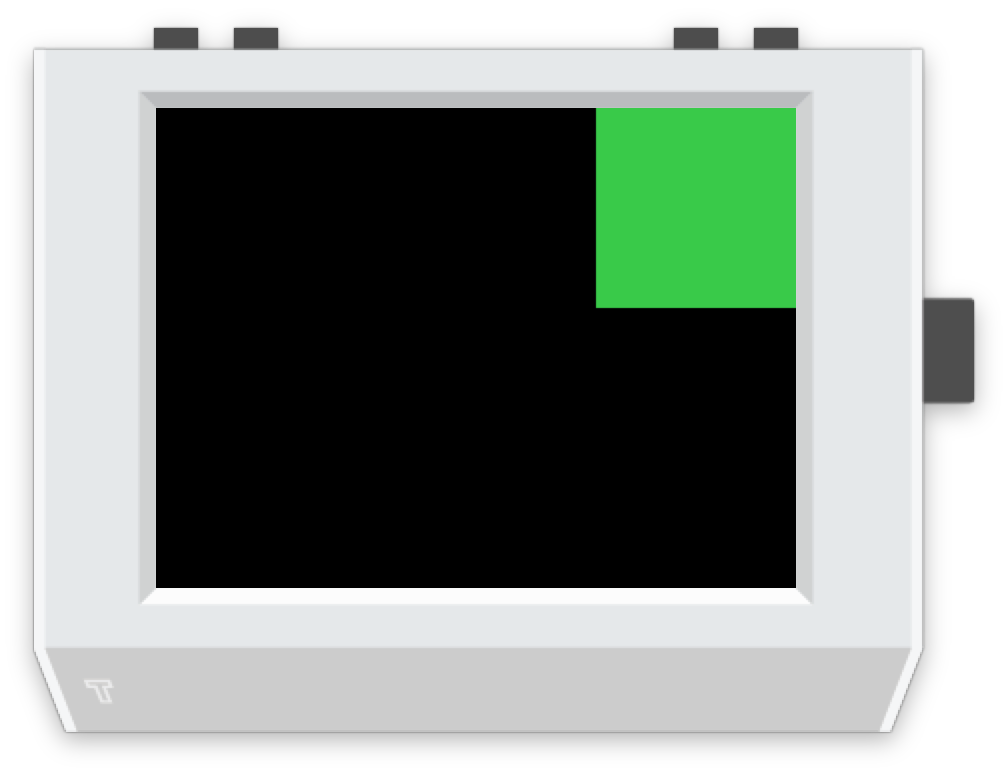`screen.rectangle(color='green', align='topright')`
left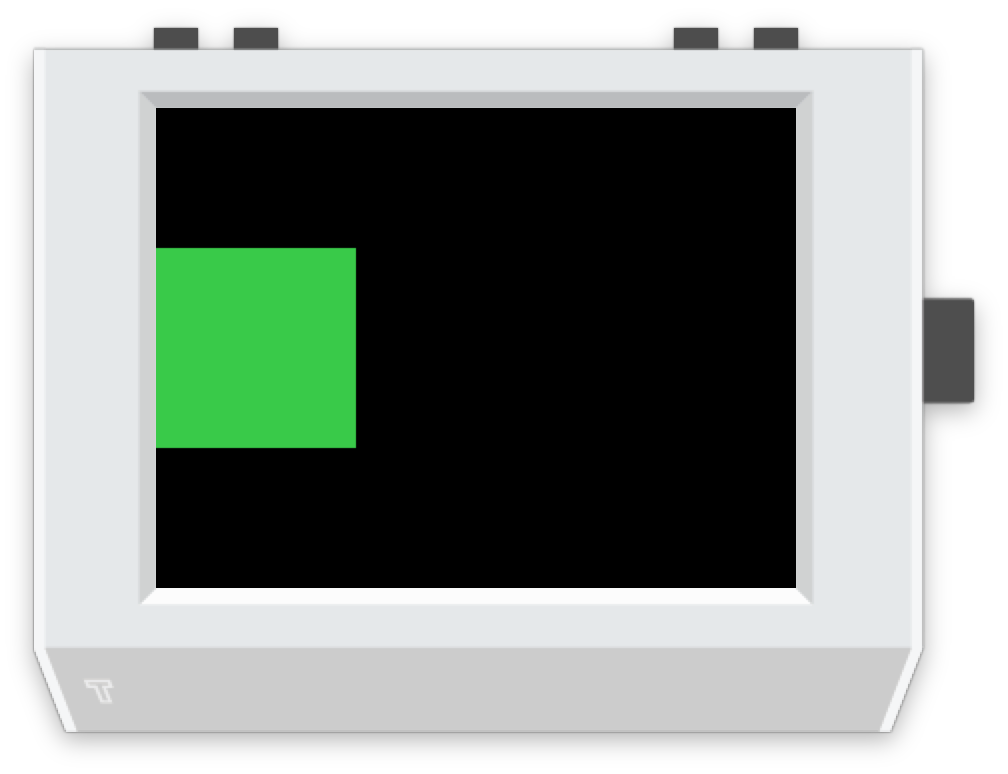`screen.rectangle(color='green', align='left')`
center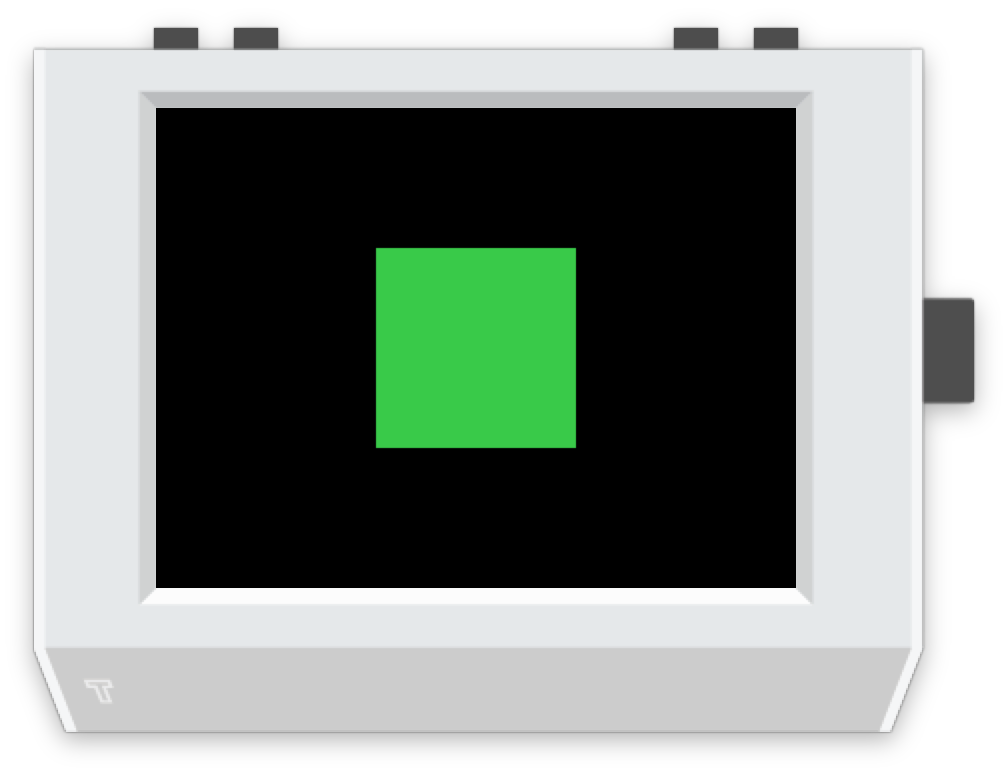`screen.rectangle(color='green', align='center')`
right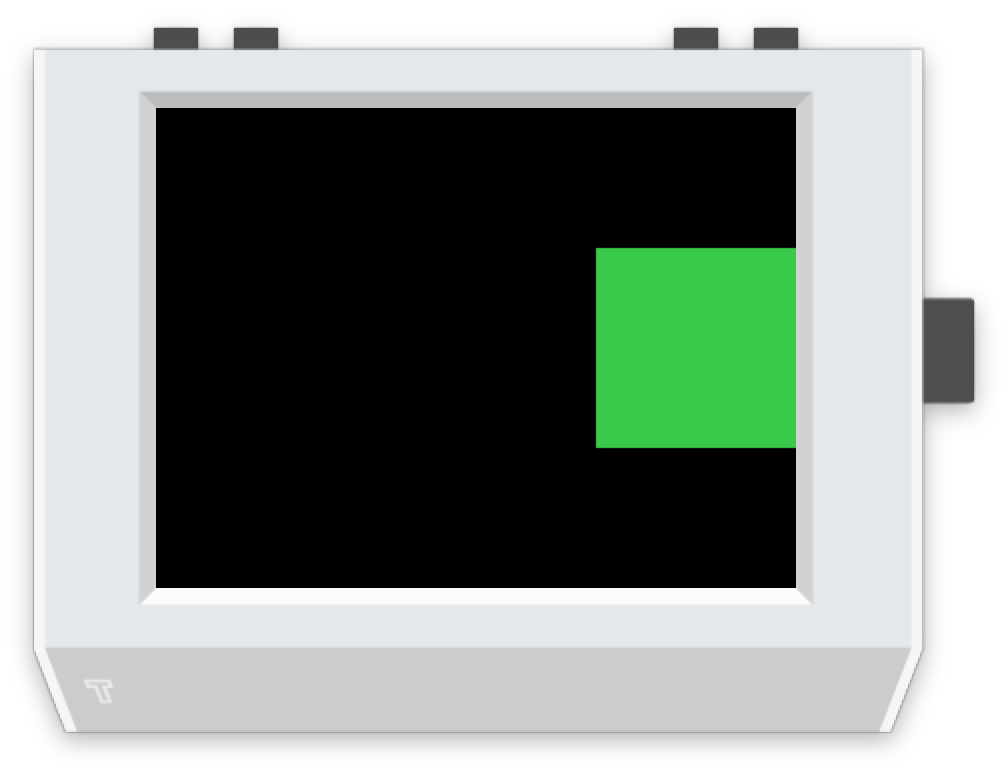`screen.rectangle(color='green', align='right')`
bottomleft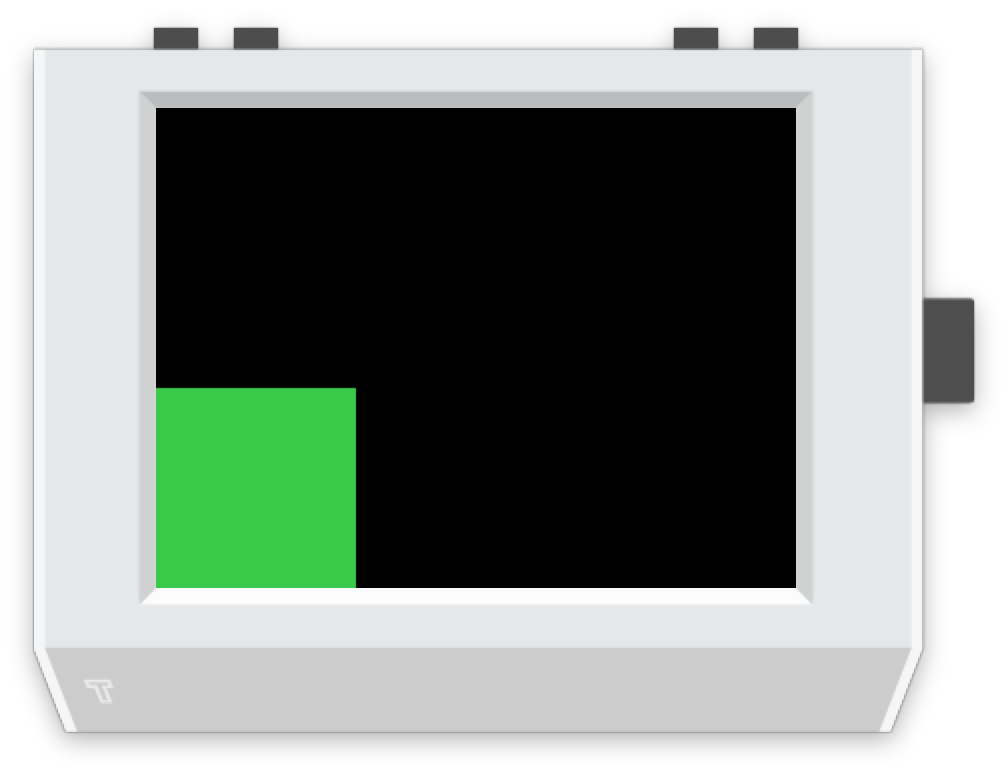`screen.rectangle(color='green', align='bottomleft')`
bottom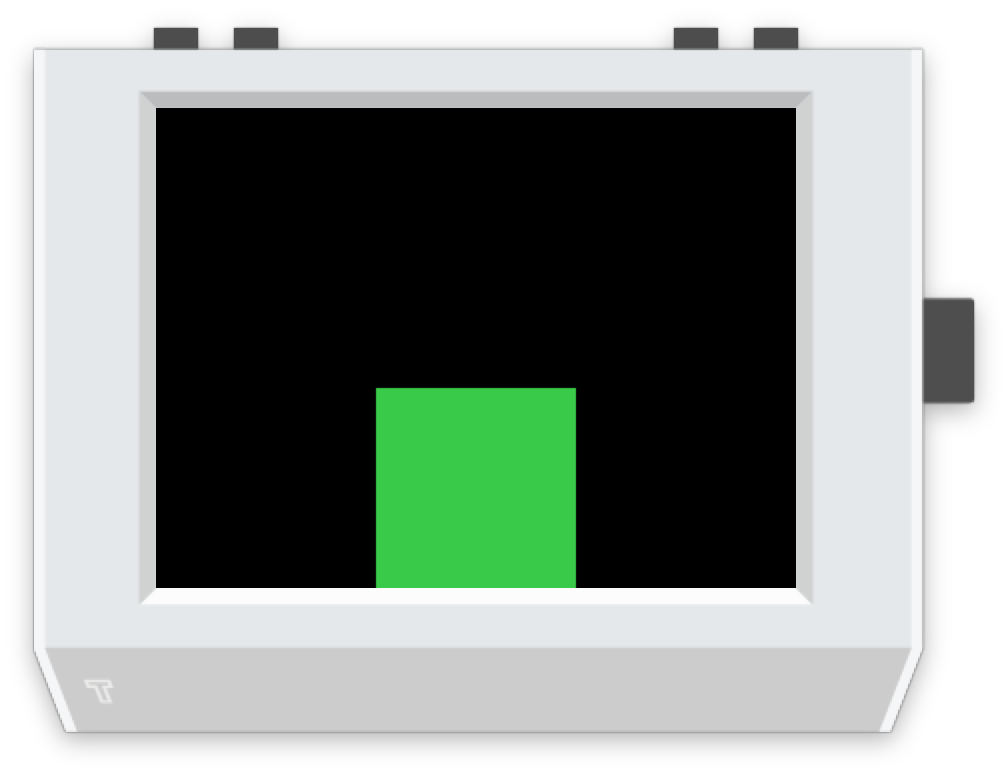`screen.rectangle(color='green', align='bottom')`
bottomright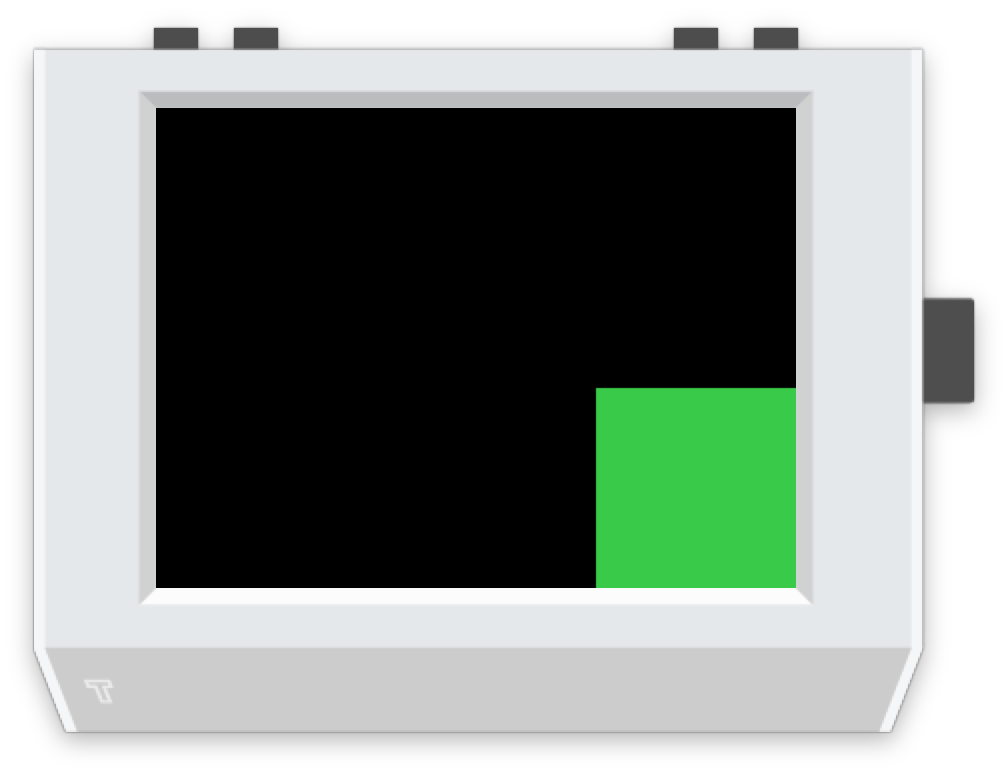`screen.rectangle(color='green', align='bottomright')`

When used with the `xy` parameter, it positions the item relative to the `xy` point.

Setting Screenshot Code
topleft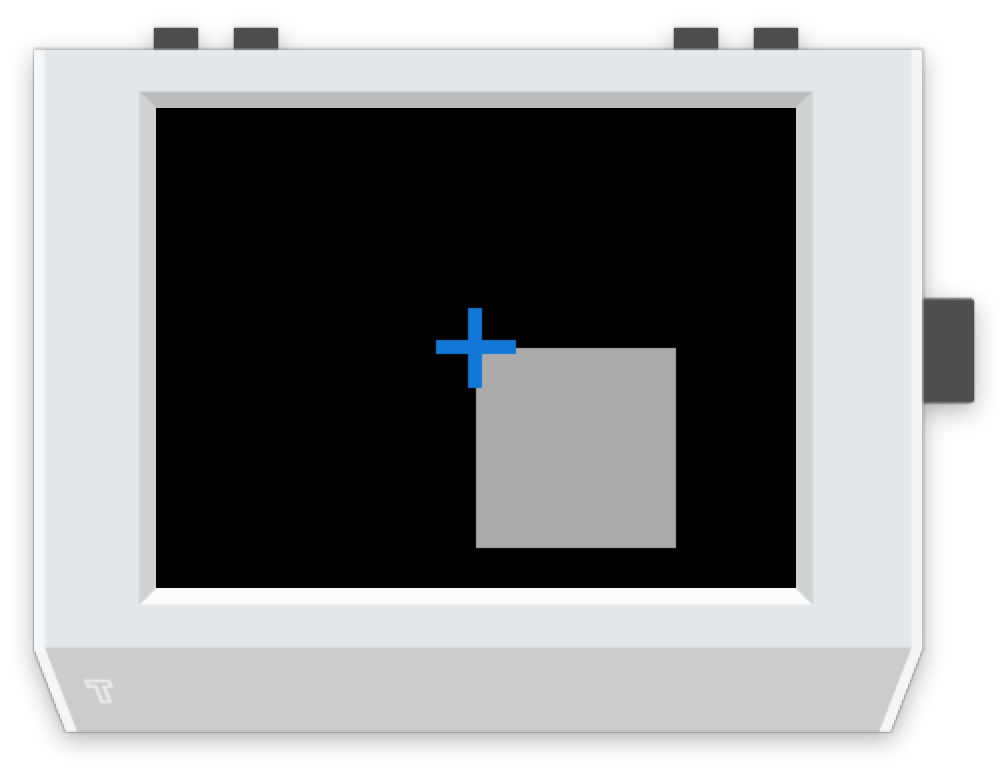`screen.rectangle(xy=(160, 120), align='topleft')`
top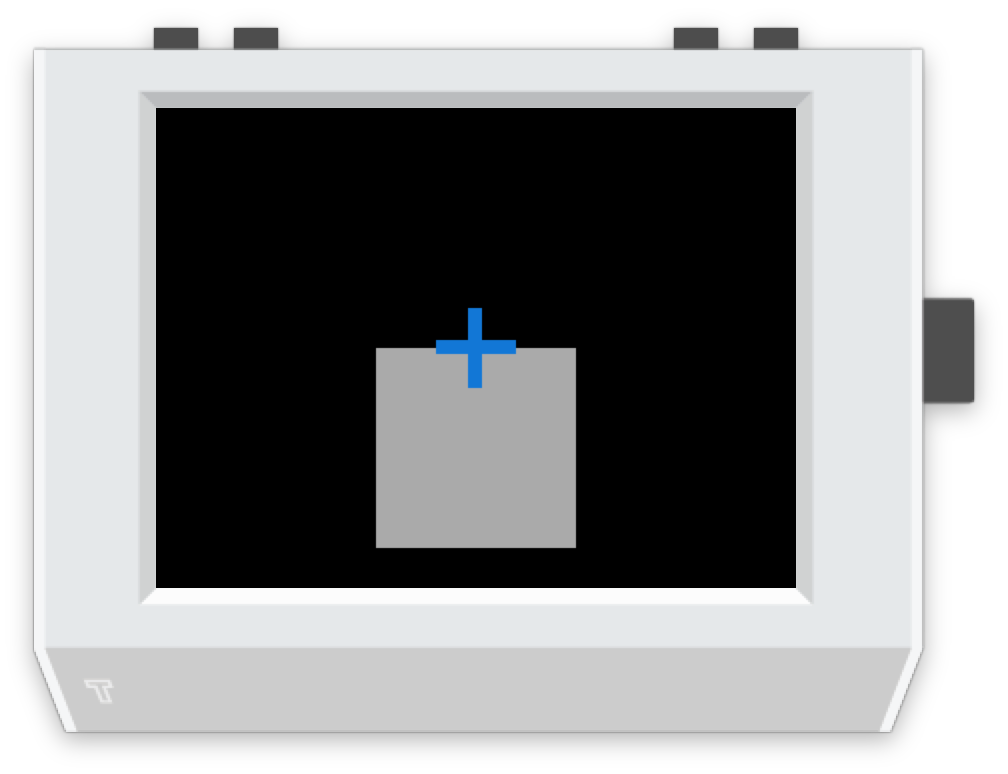`screen.rectangle(xy=(160, 120), align='top')`
topright`screen.rectangle(xy=(160, 120), align='topright')`
left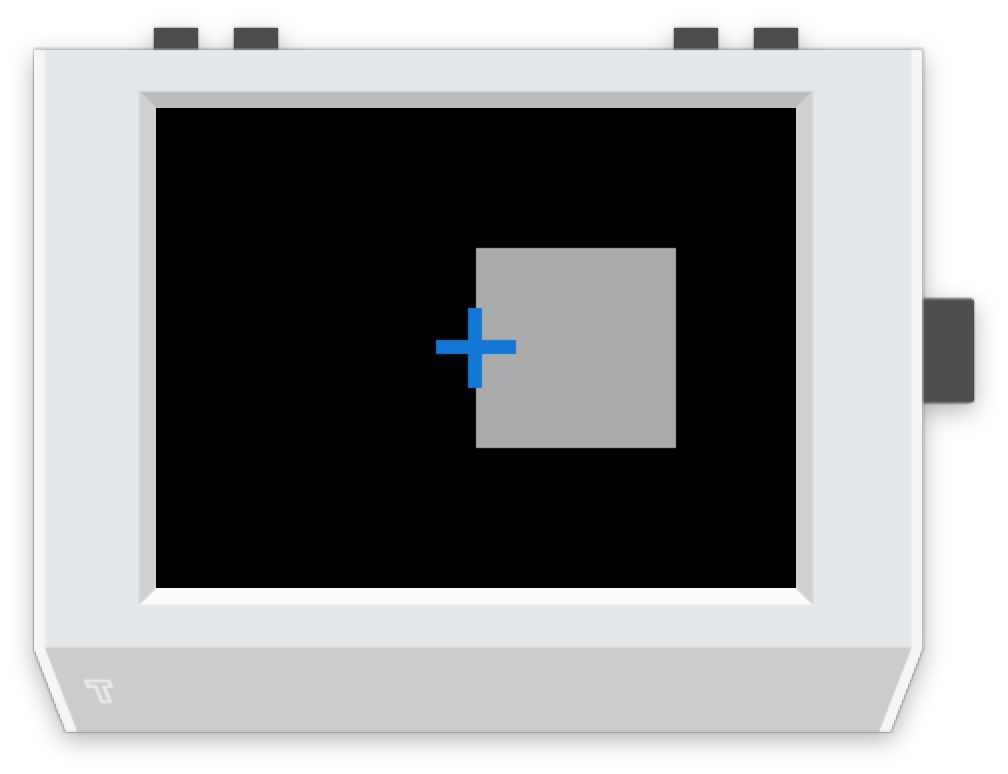`screen.rectangle(xy=(160, 120), align='left')`
center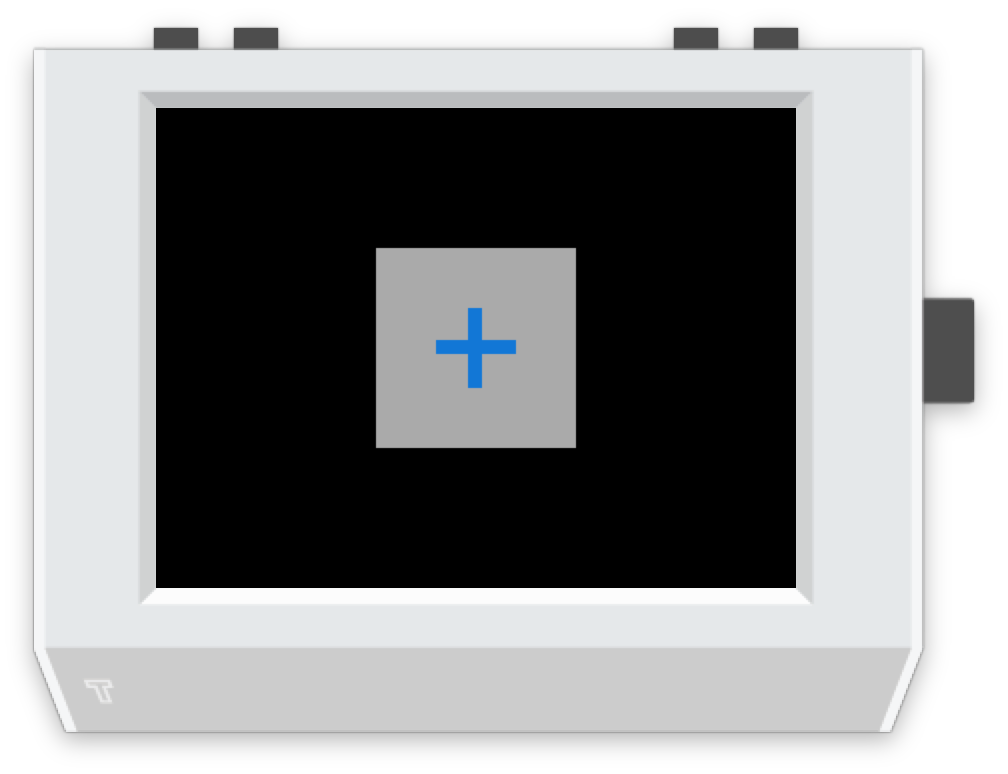`screen.rectangle(xy=(160, 120), align='center')`
right`screen.rectangle(xy=(160, 120), align='right')`
bottomleft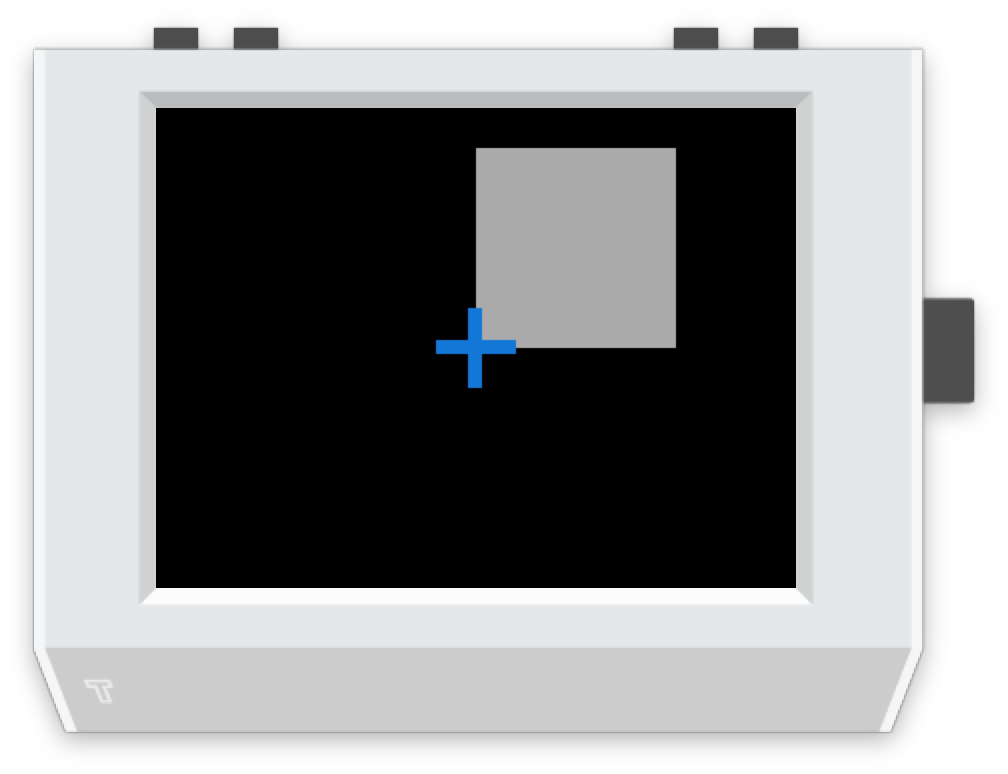`screen.rectangle(xy=(160, 120), align='bottomleft')`
bottom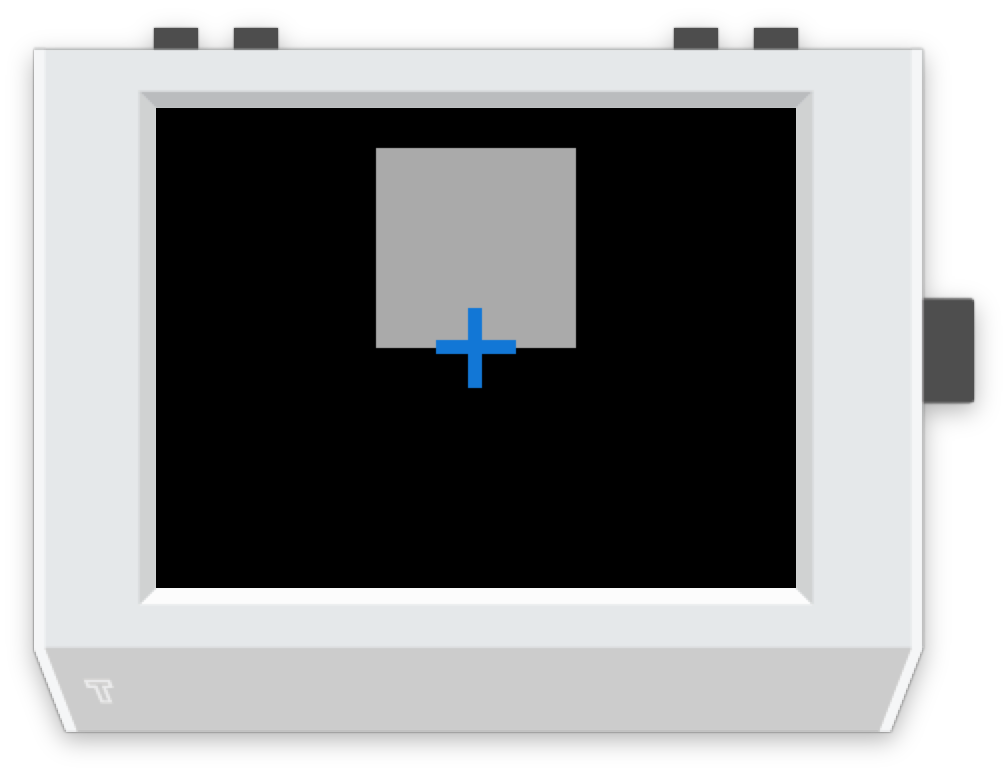`screen.rectangle(xy=(160, 120), align='bottom')`
bottomright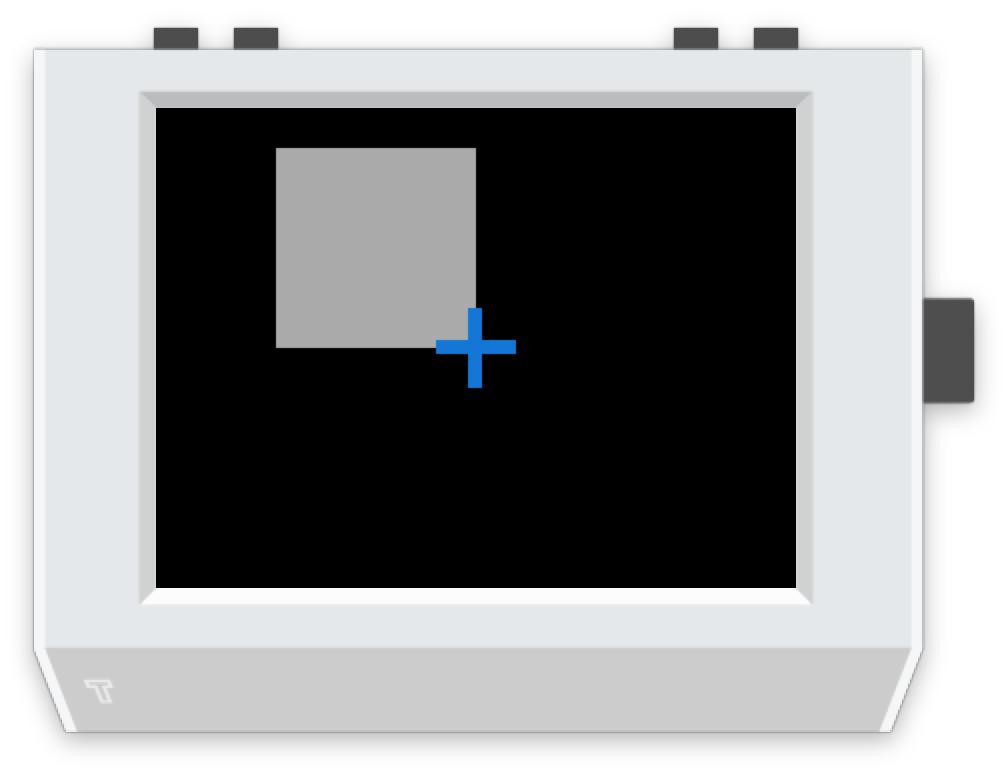`screen.rectangle(xy=(160, 120), align='bottomright')`

## The `color` option¶

The color option can be either an RGB value, or predefined color name.

### RGB values¶

RGB values (as a tuple), like `(255, 128, 0)`.

### Predefined colors¶

We also have a set of default colors, referred to by their name, as a string.

'navy' `(0, 116, 217)`
'blue' `(0, 116, 217)`
'aqua' `(127, 219, 255)`
'teal' `(57, 204, 204)`
'olive' `(61, 153, 112)`
'green' `(46, 204, 64)`
'lime' `(1, 255, 112)`
'yellow' `(255, 220, 0)`
'orange' `(255, 133, 27)`
'red' `(255, 65, 54)`
'maroon' `(133, 20, 75)`
'fuchsia' `(240, 18, 190)`
'purple' `(177, 13, 201)`
'black' `(0, 0, 0)`
'gray' `(170, 170, 170)`
'silver' `(221, 221, 221)`
'white' `(255, 255, 255)`

Thanks to http://clrs.cc for the color scheme!